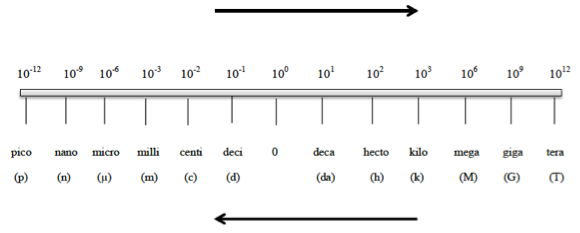# Problem: Use scientific (exponential) notation to express the following quantities in terms of the SI base units in Table 1.3:(c) 5.23 pm

###### FREE Expert Solution

We’re being asked to convert 5.23 pm to the SI base unit using scientific (exponential) notation.

Metric Prefixes are “labels” that can be placed in front of base units. Whenever using the metric prefix chart, remember that “1” is associated with the metric prefix82% (366 ratings)###### Problem Details

Use scientific (exponential) notation to express the following quantities in terms of the SI base units in Table 1.3:

(c) 5.23 pm

Frequently Asked Questions

What scientific concept do you need to know in order to solve this problem?

Our tutors have indicated that to solve this problem you will need to apply the Metric Prefixes concept. You can view video lessons to learn Metric Prefixes. Or if you need more Metric Prefixes practice, you can also practice Metric Prefixes practice problems.

What professor is this problem relevant for?

Based on our data, we think this problem is relevant for Professor O'Halloran's class at GGC.

What textbook is this problem found in?

Our data indicates that this problem or a close variation was asked in Chemistry - OpenStax 2015th Edition. You can also practice Chemistry - OpenStax 2015th Edition practice problems.